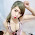Scales of intensity and magnitude

As with earthquakes, several attempts have been made to set up scales of tsunami intensity or magnitude to allow comparison between different events.

Intensity scales

The first scales used routinely to measure the intensity of tsunami were the Sieberg-Ambraseys scale, used in the Mediterranean Sea and the Imamura-Iida intensity scale, used in the Pacific Ocean. The latter scale was modified by Soloviev, who calculated the Tsunami intensity I according to the formula$\,\mathit{I} = \frac{1}{2} + \log_{2} \mathit{H}_{av}$
where Hav is the average wave height along the nearest coast. This scale, known as the Soloviev-Imamura tsunami intensity scale, is used in the global tsunami catalogues compiled by the NGDC/NOAA and the Novosibirsk Tsunami Laboratory as the main parameter for the size of the tsunami.

Magnitude scales

The first scale that genuinely calculated a magnitude for a tsunami, rather than an intensity at a particular location was the ML scale proposed by Murty & Loomis based on the potential energy. Difficulties in calculating the potential energy of the tsunami mean that this scale is rarely used. Abe introduced the tsunami magnitude scale Mt, calculated from,$\,\mathit{M}_{t} = {a} \log h + {b} \log R = \mathit{D}$
where h is the maximum tsunami-wave amplitude (in m) measured by a tide gauge at a distance R from the epicenter, a, b & D are constants used to make the Mt scale match as closely as possible with the moment magnitude scale.

1.Freebet tanpa deposit hanya ada di ANAPOKER

2.Agen judi online terbaik dan terpercaya 128AGENS

3.Agen Sportbook Terpercaya AGENS128

4.This website can live streaming , you can join at my site :
agen judi online terpercaya
Prediksi Bola

Thank you
agenpialaeropa.club
gamesonline.ga
beritasemasaterikini.net